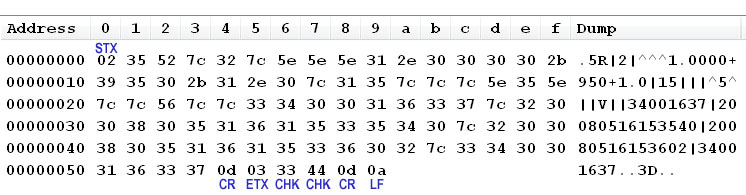# Calculate the checksum of an ASTM (or other) document on C#

When the LIS receives the ASTM message from the instrument, each segment of data includes a checksum when either the laboratory instrument or the LIS (Laboratory Information System) transmits the document.  The benefits are obvious in a mission (life) critical application to ensure what was sent is exactly what is received.

The format of an ASTM frame: <STX><Frame Data><CR><ETX><CHECKSUM 1><CHECKSUM 2><CR><LF>

The frame starts with a <STX> character (0x02) and ends with a <ETX> character (0x03), or a <ETB> if a partial frame.  Just prior to the <ETX> is a carriage return \r (no newline character), The C# escape sequences \r and \n are used purely to be able to display the string in a web page, but you will have to replace the  \r  with an ASCII 13 or HEX 0D. In addition the trailing \r\n characters must be replaced with ASCII 13 and ASCII 10 or HEX 0D 0A. That being said you can run the string above through the C# method shown below and the escape sequences will be translated correctly.

All of the characters AFTER the <STX> and up to and including the <ETX> (or <ETB>) character is the data used to calculate the checksum for this message.  The following C# method calculates the checksum by adding the ASCII values of each character in the string and then performing a mod 256 calculation on the total value for all characters in the ASTM frame.  The checksum is then appended to the end of the message, and in this case the value is 3D. Remember the checksum is displayed as a 2 byte HEX string of characters.The maximum character limit for a frame in ASTM is 247 characters (including overhead). If a given message is less than 240 characters, it is sent in an end frame with a <ETX>, checksum, and a <CR><LF>. If a message is greater than 240 characters, the message is sent in intermediate frames containing this sequence <ETB>, checksum, and a <CR><LF>.

## First Step: include T class:

```public class T
{
public const byte ENQ = 5;
public const byte ACK = 6;
public const byte NAK = 21;
public const byte EOT = 4;
public const byte ETX = 3;
public const byte ETB = 23;
public const byte STX = 2;
public const byte NEWLINE = 10;
public static byte[] ACK_BUFF = { ACK };
public static byte[] ENQ_BUFF = { ENQ };
public static byte[] NAK_BUFF = { NAK };
public static byte[] EOT_BUFF = { EOT };
}```

## Last Step: calculate the checksum

```/// <summary>
/// Reads checksum of an ASTM frame, calculates characters after STX,
/// up to and including the ETX or ETB. Method assumes the frame contains an ETX or ETB.
/// </summary>
/// <param name="frame">frame of ASTM data to evaluate</param>
/// <returns>string containing checksum</returns>
public string GetCheckSumValue(string frame)
{
string checksum = "00";

int byteVal = 0;
int sumOfChars = 0;
bool complete = false;

//take each byte in the string and add the values
for (int idx = 0; idx < frame.Length; idx++)
{
byteVal = Convert.ToInt32(frame[idx]);

switch (byteVal)
{
case T.STX:
sumOfChars = 0;
break;
case T.ETX:
case T.ETB:
sumOfChars += byteVal;
complete = true;
break;
default:
sumOfChars += byteVal;
break;
}

if (complete)
break;
}

if (sumOfChars > 0)
{
//hex value mod 256 is checksum, return as hex value in upper case
checksum = Convert.ToString(sumOfChars % 256, 16).ToUpper();
}

//if checksum is only 1 char then prepend a 0
return (string)(checksum.Length == 1 ? "0" + checksum : checksum);
}```Author